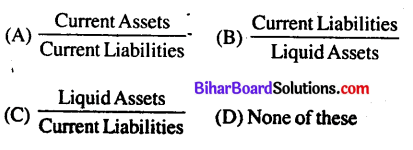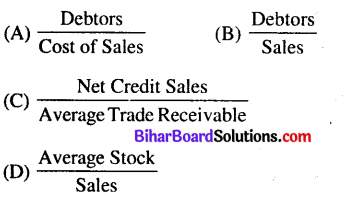# Bihar Board 12th Accountancy Objective Answers Chapter 10 Accounting Ratios

Bihar Board 12th Accountancy Objective Questions and Answers

## Bihar Board 12th Accountancy Objective Answers Chapter 10 Accounting Ratios

Question 1.
The ideal liquid ratio is :
(A) 2:1
(B) 1:1
(C) 5:1
(D) 4:1
(B) 1:1Question 2.
The ideal current ratio is :
(A) 2 : 1
(B) 1 : 2
(C) 3 : 2
(D) 3 :4
(A) 2 : 1

Question 3.
Operating Ratio is:
(A) Profitability Ratio
(B) Activity Ratio
(C) Solvency Ratio
(D) None of these
(A) Profitability Ratio

Question 4.
Profitability Ratio is generally shown in :
(A) Simple Ratio
(B) Percentage
(C) Times
(D) None of these
(B) PercentageQuestion 5.
If sales is 7 4,20,000 sales returns is 7 20,000 and cost of goods sold 7 3,20,000 gross profit ratio will be :
(A) 20%
(B) 25%
(C) 15%
(D) 10%
(A) 20%

Question 6.
Stock turnover ratio comes under :
(A) Liquidity Ratio
(B) Profitability Ratio
(C) Activity Ratio
(D) None of these
(C) Activity Ratio

Question 7.
The satisfactory ratio between internal and external equity is. :
(A) 1 :2
(B) 2 :1
(C) 3 : 1
(D) 4:1
(B) 2 :1

Question 8.
Current Ratio includes:
(A) Stock
(B) Debtors
(C) Cash
(D) All of these
(C) Cash

Question 9.
Current Ratio =
(A) Current Assets/Current Liabilities
(B) Liquid Assets/Current Liabilities
(C) Liquid Assets/Current Assets
(D) Fixed Assets/Current Assets
(A) Current Assets/Current Liabilities

Question 10.
Liquid Assets include :
(A) Bills Receivable
(B) Debtors
(C) Cash Balance
(D) All of these
(D) All of theseQuestion 11.
Which of the following assets is not taken into consideration in calculating acid-test ratio ?
(A) Cash
(B) Bills Receivable
(C) Stock
(D) None of these
(C) Stock

Question 12.
When Cash is 7 10,000 Stock is 7 25,000, B/R is 7 5,000 Creditors is 7 22,000 and Bank Overdraft is 7 8,000 then current ratio is :
(A) 2 : 1
(B) 4 : 3
(C) 3 : 4
(D) 1 : 2
(B) 4 : 3

Question 13.
The two basic measures of liquidity are :
(A) Inventory Turnover and Current Ratio
(B) Current Ratio and Liquid Ratio
(C) Current Ratio and Averge Collection Period
(D) Current Ratio and Debtors Turnover Ratio
(B) Current Ratio and Liquid Ratio

Question 14.
Liquidity Ratio:(C)

Question 15.
The term ‘Current Liabilities’ does not include: .
(A) Sundry Creditors
(B) Debentures
(C) Bills Payable
(D) Outstanding Expenses
(B) Debentures

Question 16.
The term‘Current Assets’include
(A) Long-term Investment
(B) Short-term Investment
(C) Furniture
(D) Preliminary Expenses
(B) Short-term InvestmentQuestion 17.
Liquid Ratio is also known as:
(A) Current Ratio
(B) Quick Ratio
(C) Capital Ratio
(D) None of these
(B) Quick Ratio

Question 18.
To test the liquidity of a concern which of the following ratios is useful ?
(A) Capital Tunover Ratio
(B) Acid Test Ratio
(C) Stock Turnover Ratio
(D) Net Profit Ratio
(B) Acid Test Ratio

Question 19.
Which of the following transactions will improve the current ratio ?
(A) Purchase of good for cash
(C) Payment of creditors
(D) Credit purchase of goods
(C) Payment of creditors

Question 20.
Debt-equity ratio is :
(A) Liquidity Ratio
(B) Activity Ratio
(C) Solvency Ratio
(D) Operating Ratio
(C) Solvency Ratio

Question 21.
The formula for finding out Debt-Equity Ratio is:
(A) Long-term Debts/Shareholders’ Funds
(B) Debentures/Equity Capital
(C) Net Profit/Total Capital
(D) None of these
(A) Long-term Debts/Shareholders’ Funds

Question 22.
The formula for ascertaining Total Assets to Debt Ratio is:(A)Question 23.
Proprietory Ratio indicates the relationship between proprietor’s funds and….
(A) Reserve
(B) Share Capital
(C) Total Assets
(D) Debentures
(C) Total Assets

Question 24.
Proprietory ratio is calculated by the following formula:(C)

Question 25.
Which one of the following ratios is most important in determining the long-term solvency of a company ?
(A) Profitability Ratio
(B) Debt-Equity Ratio
(C) Stock Turnover Ratio
(D) Current Ratio
(B) Debt-Equity Ratio

Question 26.
Total Assets ₹ 8,10,000
Total Liabilities ₹ 2,60,000
Current Liabilities ₹ 40,000
Debt-equity ratio is:
(A) 0.05:1
(B) 0.4:1
(C) 2.5:1
(D) 4:1
(C) 2.5:1

Question 27.
Equity share capital ₹ 15,00,000
Reserve and Surplus ₹ 7,50,000
Total Assets ₹ 45,00,000
Properletory Ratio ?
(A) 50%
(B) 33.3%
(C) 200%
(D) 60%
(A) 50%Question 28.
Total Assets ₹ 7,70,000
Total Liabilities ₹ 2,60,000
Current Liabilities ₹ 40,000
Total Assets to Debt Ratio is:
(A) 3.5 : 1
(B) 2.56 : 1
(C) 2.8 :1
(D) 3:1
(A) 3.5 : 1

Question 29.
Profitability Ratios are generally expressed in :
(A) Simple Ratio
(B) Percentage
(C) Times
(D) None of these
(B) Percentage

Question 30.
The ratios are primarily measures of earning capacity of the business.
(A) Liquidity
(B) Activity
(C) Debt
(D) Profitability
(D) Profitability

Question 31.
The gross profit ratio is the ratio of gross profit to :
(A) Net Cash Sales
(B) Net Credity Sales
(C) Closing Stock
(D) Net Total Sales
(D) Net Total Sales

Question 32.
Operating Ratio is:
(A) Profitability Ratio
(B) Activity Ratio
(C) Solvency Ratio
(D) None of these
(A) Profitability RatioQuestion 33.
Which of the following is an operating’ income ?
(A) Sale of Merchandise
(B) Interest Income
(C) Dividend Income
(D) Profit on the sale of old car
(A) Sale of Merchandise

Question 34.
Which of the following non-operating expense?
(A) Rent
(B) Selling Expenses
(C) Wages
(D) Loss on Sale of Machinery
(D) Loss on Sale of Machinery

Question 35.
The following groups of ratios primarily measure risk
(A) Liquidity, activity and profitability
(B) Liquidity, activity and common stock
(C) Liquidity, activity and debt
(D) Activity, debt and profitability
(D) Activity, debt and profitability

Question 36.
To know the return on investment, by capital employed we mean:
(A) Net Fixed Assets
(B) Current Asset-Current Liabilities
(C) Gross Block
(D) Fixed Assets + Current Assets-Current Liabilities
(D) Fixed Assets + Current Assets-Current Liabilities

Question 37.
The term fixed assets include :
(A) Cash
(B) Machinery
(C) Debtors
(D) Prepaid Expenses
(B) MachineryQuestion 38.
Ratio based on figures of profit & loss as well a the Balance sheet are:
(A) Profitability Ratios
(B) Operation Ratio
(C) Liquidity Ratio
(D) Composite Ratio
(D) Composite Ratio

Question 39.
Debtors Turnover Ratio :(C)

Question 40.
When opening stock is ₹ 50,000 closing stock ₹ 60,000 and cost of goods sold is ₹ 2,20,000, then stock turn over ratio is:
(A) 2 times
(B) 3 times
(C) 4 times
(D) 5 times
(A) 2 times

Question 41.
What does Creditors Turnover Ratio take into account:
(A) Total credit purchases
(B) Total credit sales
(C) Total cash sales
(D) Total cash purchases